Build Wavelet Tree Objects

The following sections explain how to extend the toolbox with new objects through four examples.

Building a Wavelet Tree Object (WTREE)

This example creates a new class of objects: WTREE.

Starting from the class DTREE and overloading the methods split and merge, we define a wavelet tree class.

To plot a WTREE, the DTREE plot method is used.

The definition of the new class is described below.

Class WTREE (parent class: DTREE)

Fields

 dtree Parent object dwtMode DWT extension mode wavInfo Structure (wavelet information)

wavInfo Structure information

 wavName Wavelet Name Lo_D Low Decomposition filter Hi_D High Decomposition filter Lo_R Low Reconstruction filter Hi_R High Reconstruction filter

Methods

 wtree Constructor for the class WTREE. merge Merge (recompose) the data of a node. split Split (decompose) the data of a terminal node.

Working With Wavelet Tree Objects (WTREE)

1-D Object

x = noisbloc;

Define the level and the wavelet.

lev = 3;
wav = 'db2';

Create the wavelet tree.

t = wtree(x,lev,wav);

Plot the wavelet tree. The approximations are labeled in yellow and the details are labeled in red. The detail nodes cannot be split.

plot(t)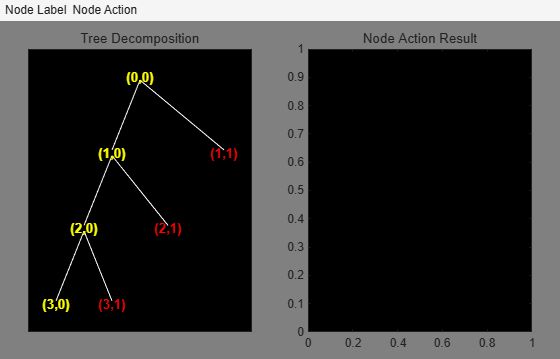Change Node Label from Visualize to Split-Merge, and then click node (7), to get this figure: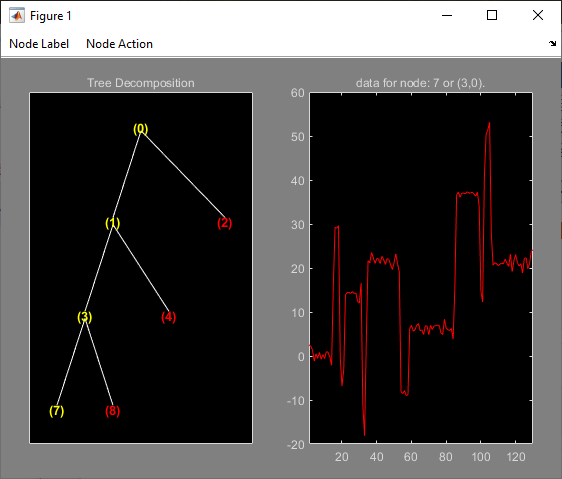Change Node Action from Visualize to Split-Merge, and merge node (3). Then change Node Action from Split-Merge to Visualize, and click node (3) to get this figure: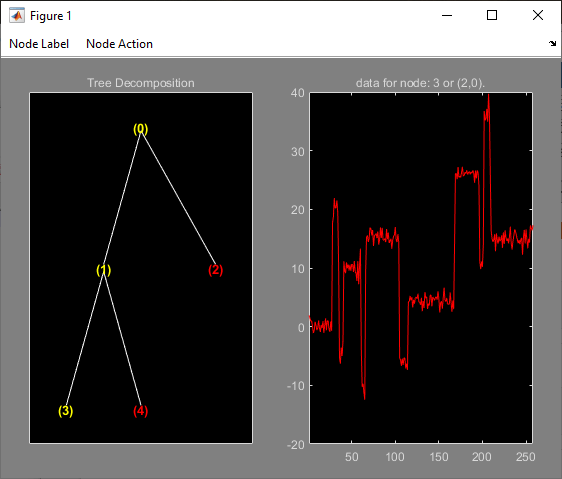2-D Object

Define the level and the wavelet.

lev = 2;
wav = 'db2';

Create the wavelet tree.

t = wtree(X,lev,wav);

Plot the tree.

plot(t)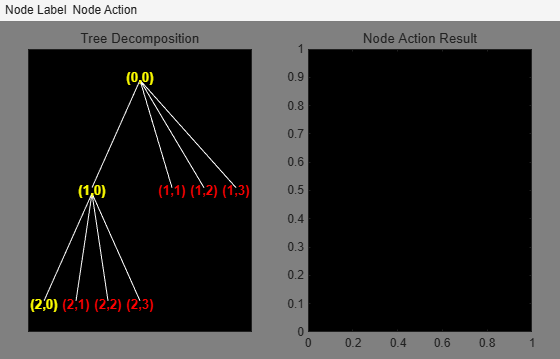Change Node Label from Depth_Position to Index. Click the node (5). You get the following plot.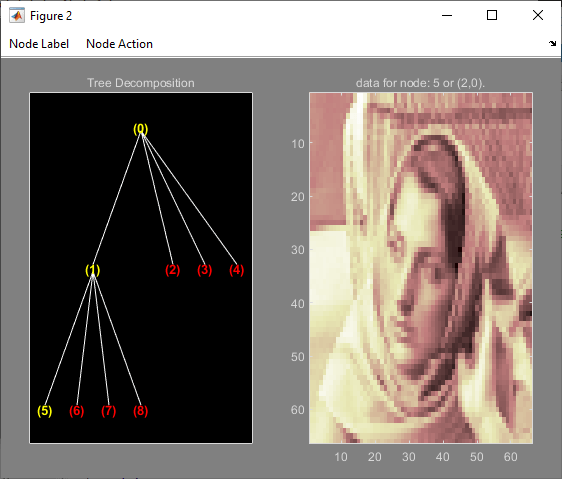Click the node (2). You obtain the following plot.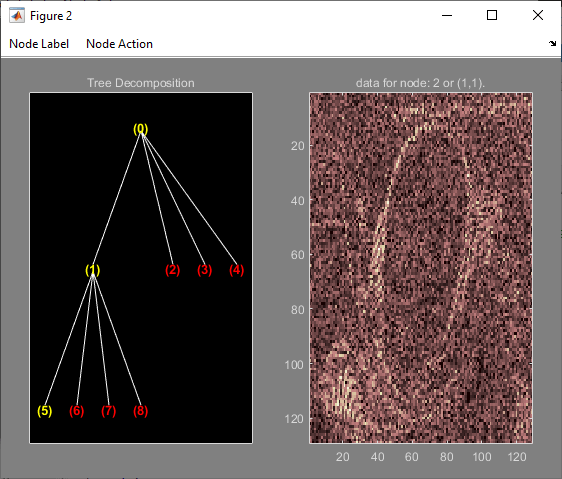Change Node Action from Visualize to Split-Merge. Split the node (5). Change Node Action from Split-Merge to Visualize. Click the node (21). You obtain the following plot.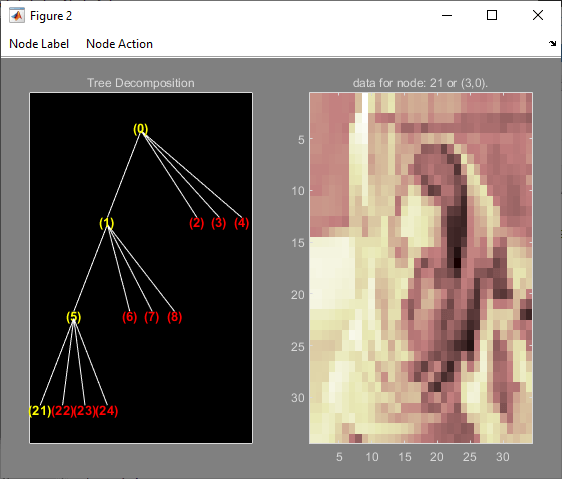Building a Right Wavelet Tree Object (RWVTREE)

This example creates a new class of objects: RWVTREE.

We define a right wavelet tree class starting from the class WTREE and overloading the methods split, merge, and plot (inherited from DTREE).

The plot method shows how to add Node Labels.

The definition of the new class is described below.

Class RWVTREE (parent class: WTREE)

Fields

 dummy Not used wtree Parent object

Methods

 rwvtree Constructor for the class RWVTREE. merge Merge (recompose) the data of a node. plot Plot RWVTREE object. split Split (decompose) the data of a terminal node.

Working With Right Wavelet Tree Objects (RWVTREE)

1-D Object

x = noisbloc;

Define the level and the wavelet.

lev = 3;
wav = 'db2';

Create the wavelet tree.

t = rwvtree(x,lev,wav);

Plot the tree. The approximations are labeled in yellow and the details are labeled in red. The detail nodes cannot be split.

plot(t)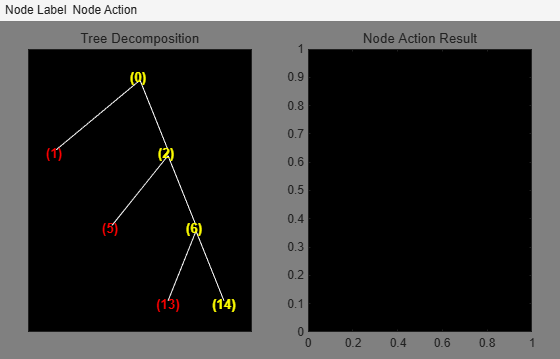Change Node Action from Visualize to Split-Merge. Merge the node (6). Change Node Action from Split-Merge to Visualize. Click the node (6). You obtain the following plot.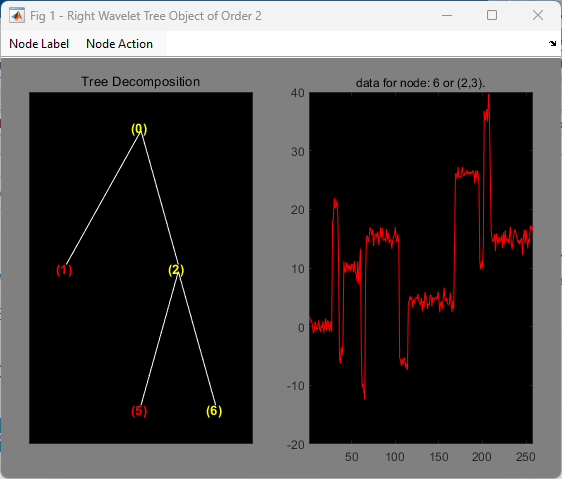2-D Object

Define the level and the wavelet.

lev = 2;
wav = 'db2';

Create the wavelet tree.

t = wtree(X,lev,wav);

Plot the tree.

plot(t)Click the node (2,0). You get the following plot.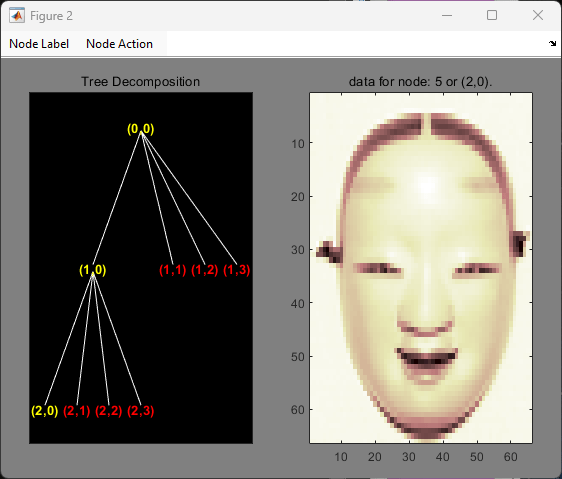Change Node Action from Visualize to Split-Merge. Split the node (2,0). Change Node Action from Split-Merge to Visualize. Click the node (3,0). You obtain the following plot.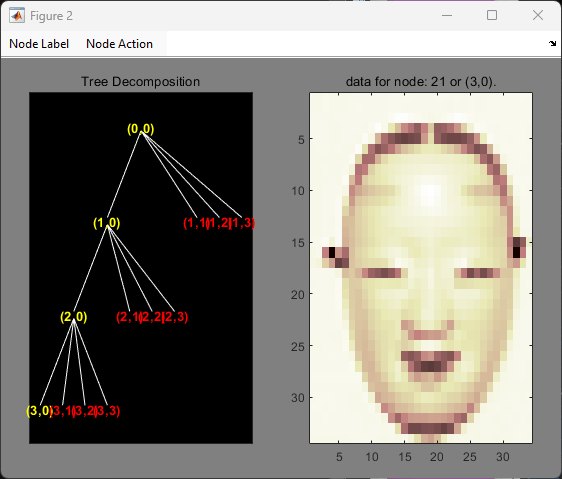Building a Wavelet Tree Object (WVTREE)

This example creates a new class of objects: WVTREE.

We define a wavelet tree class starting from the class WTREE and overloading the methods get, plot, and recons (all inherited from DTREE).

The split and merge methods of the class WTREE are used.

The plot method shows how to add Node Labels and Node Actions.

The definition of the new class is described below.

Class WVTREE (parent class: WTREE)

Fields

 dummy Not used wtree Parent object

Methods

 wvtree Constructor for the class WVTREE. get Get WVTREE object field contents. plot Plot WVTREE object. recons Reconstruct node coefficients.

Working With Wavelet Tree Objects (WVTREE)

1-D Object

x = noisbloc;

Define the level and the wavelet.

lev = 3;
wav = 'db2';

Create the wavelet tree.

t = wvtree(x,lev,wav);

Plot the tree. The approximations are labeled in yellow and the details are labeled in red. The detail nodes cannot be split.

plot(t)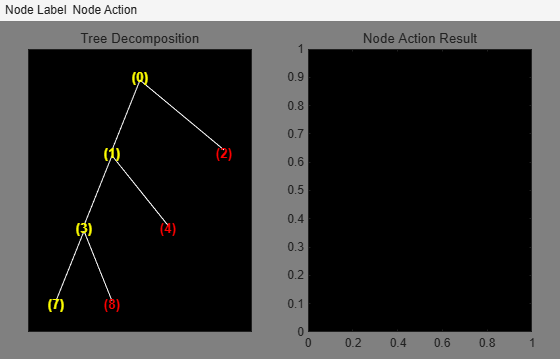Change Node Action from Visualize to Split-Merge. Merge the node (3). Change Node Action from Split-Merge to Reconstruct. Click the node (3). You obtain the following plot.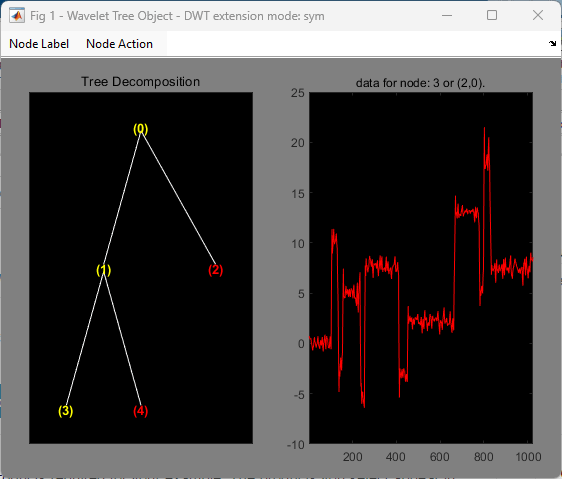2-D Object

Define the level and the wavelet.

lev = 2;
wav = 'db1';

Create the wavelet tree.

t = wvtree(X,lev,wav);

Plot the tree.

plot(t)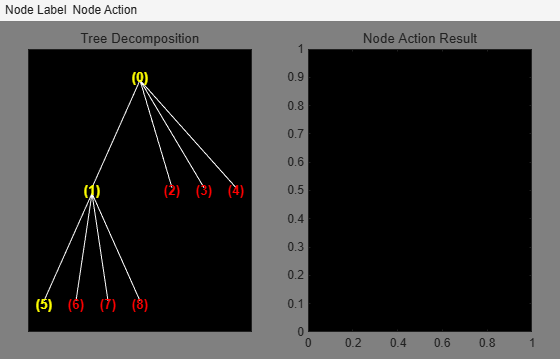Click the node (5). You get the following plot.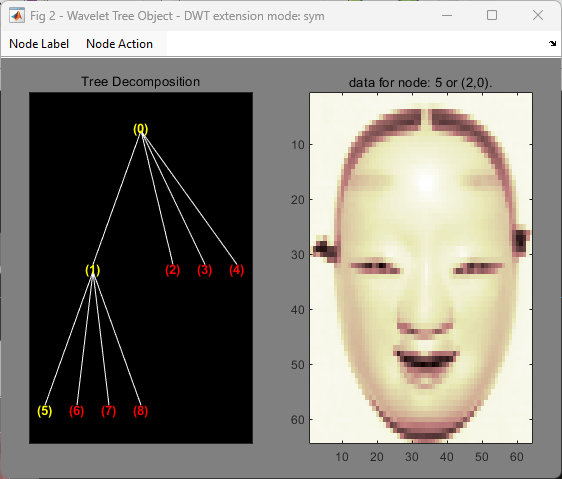Click the node (2). You obtain the following plot.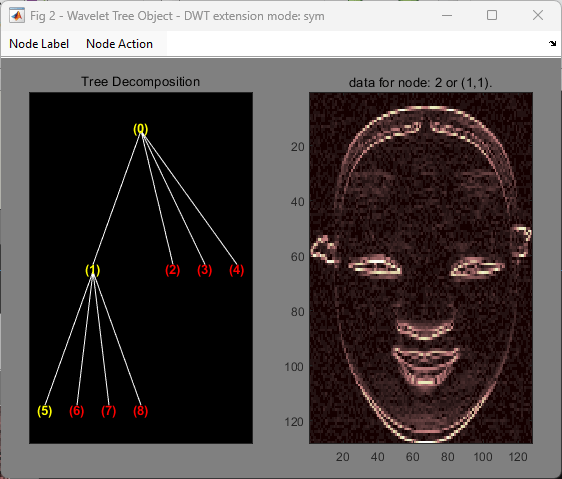Change Node Action from Visualize to Split-Merge. Split the node (5). Change Node Action from Split-Merge to Reconstruct. Click the node (21). You obtain the following plot.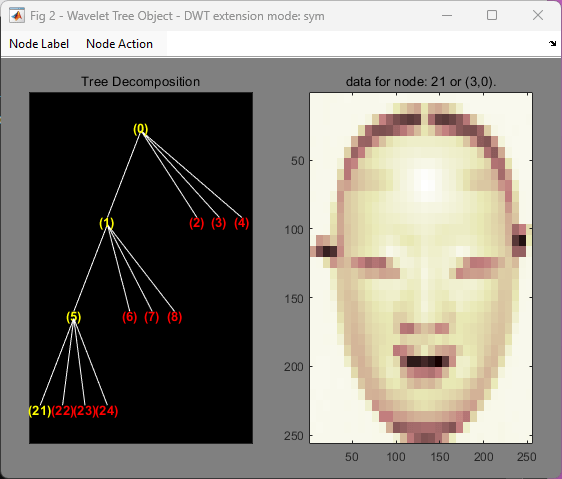Building a Wavelet Tree Object (EDWTTREE)

This example creates a new class of objects: EDWTTREE.

We define an ε-DWT tree class starting from the class DTREE and overloading the methods merge, plot, recons, and split.

The plot method shows how to add Node Labels, Node Actions, and Tree Actions.

The definition of the new class is described below.

Class EDWTTREE (parent class: DTREE)

Fields

 dtree Parent object dwtMode DWT extension mode wavInfo Structure (wavelet information)

Fields Description

wavInfo

 wavName Wavelet Name Lo_D Low Decomposition filter Hi_D High Decomposition filter Lo_R Low Reconstruction filter Hi_R High Reconstruction filter

Methods

 edwttree Constructor for the class EDWTTREE. merge Merge (recompose) the data of a node. plot Plot EDWTTREE object. recons Reconstruct node coefficients. split Split (decompose) the data of a terminal node.

Working With Wavelet Tree Object (EDWTTREE)

x = noisbloc;

Define the level and the wavelet.

lev = 2;
wav = 'haar';

Create the wavelet tree.

t = edwttree(x,lev,wav);

Plot the tree. The approximations are labeled in yellow and the details are labeled in red. The detail nodes cannot be split. The title of the figure contains the DWT extension mode used ('per' in the present example) and the name of the denoising method.

plot(t)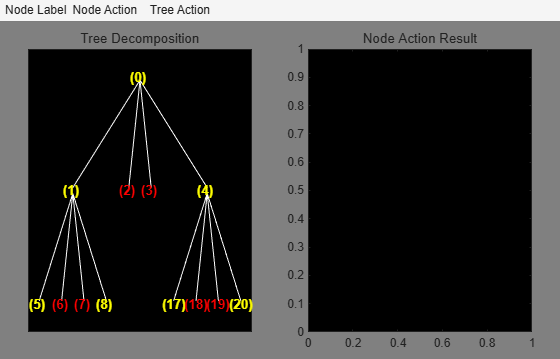Click the node (0). You obtain the following plot.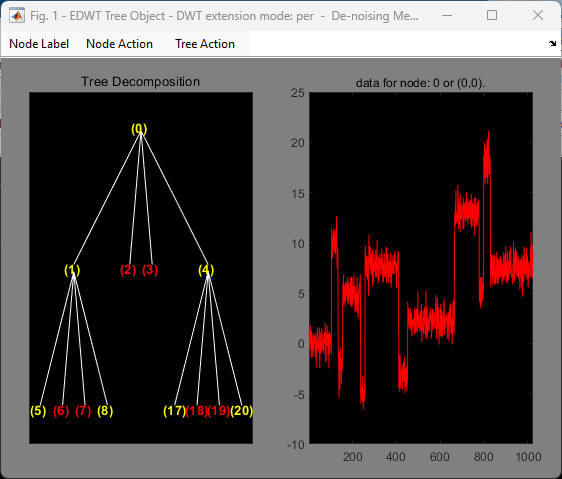Change Node Action from Visualize to Split-Merge. Split the nodes (5) and (20).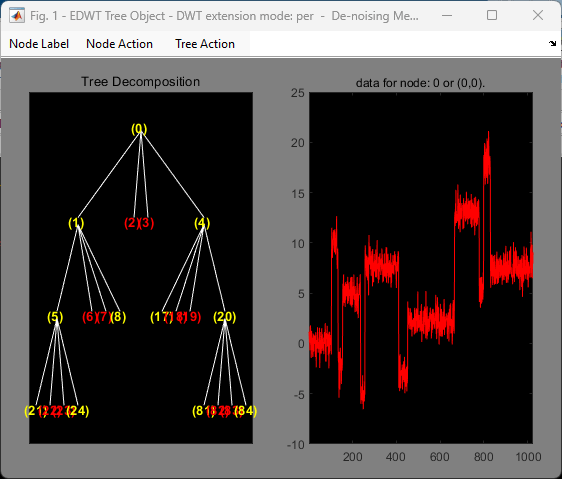Select Tree Action: De-noise. Click the node (0). You obtain the following plot.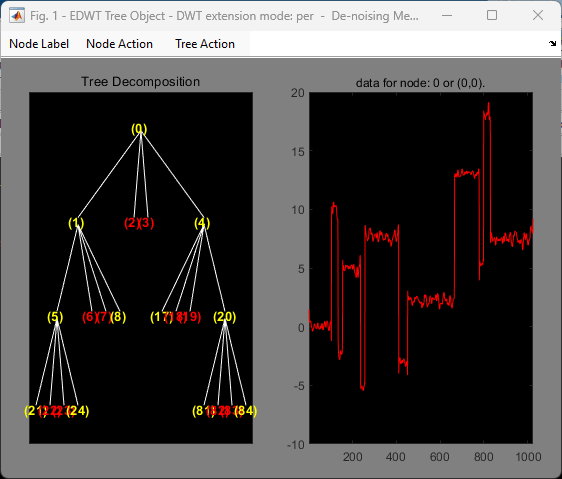Wavelet Toolbox DocumentationGet trial now